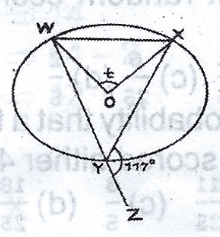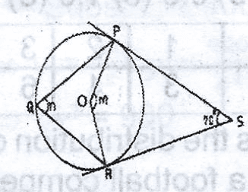# Mathematics 2018 Past Questions | WAEC

Study the following Mathematics past questions and answers for JAMBWAEC  NECO and Post-JAMB. Get prepared with official past questions and answers for upcoming examinations.

31.In the diagram, which of the following ratios is equal to $$\frac{|PN|}{|PQ|}$$?

• A.$$\frac{|PN|}{|PR|}$$
• B. $$\frac{|PM|}{|PQ|}$$
• C. $$\frac{|PM|}{|PR|}$$
• D. $$\frac{|PR|}{|PQ|}$$

32. If P and Q are two statements, under what condition would p|q be false?

• A. If p is true and q is true
• B. If p is true and q is false
• C. If p is false and q is false
• D. If p is false and q is true

33. Find the median of 2, 1, 0, 3, 1, 1, 4, 0, 1 and 2

• A. 0.0
• B. 0.5
• C. 1.0
• D. 1.5

34. Find the mean deviation of 20, 30, 25, 40, 35, 50, 45, 40, 20 and 45

• A. 8
• B. 9
• C. 10
• D.

35.Find the value of t in the diagram

• A. 63º
• B. 117º
• C. 126º
• D. 234º

36.In the diagram, PR is a tangent to the circle at Q, QT//RS,

• A. 40
• B. 65
• C. 85
• D. 95

37.In the diagram, WXYZ is a rectangle with dimension 8cm by 6cm. P, Q, R and S are the midpoints of the sides of the rectangle as shown. Using this information, what type of quadrilateral is the shaded region?

• A. Trapezium
• B. Prism
• C. Rectangle
• D. Rhombus

38.In the diagram, PS and RS are tangents to the circle centre O,

• A. 110 ${}^{o}$
• B. 135 ${}^{o}$
• C. 165 ${}^{o}$
• D. 225 ${}^{o}$

39.In the diagram, WXYZ is a rectangle with diamension 8cm by 6cm. P, Q, R and S are the midpoints of the rectangle as shown. Using this information calculate the area of the part of the rectangle that is not shaded

• A. 25cm
• B. 24cm
• C. 16cm
• D. 12cm

40. The solution of x + 2 $\ge$ 2x + 1 is illustrated

• A. i
• B. ii
• C. iii
• D. iv
Scroll to Top